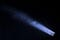# Hyper-parameter OptimizationPhoto by Paul Green on Unsplash

If you were to count all the possible classification algorithms and their parameters available just within the sklearn API, you would end up with something like 1.2 duodecillion combinations (okay, I don’t actually know, but a s*** ton). Each combination is something that we might want to try in our effort to find the best performing model for our problem. There is no free lunch after all. Data Scientists call this search hyperparameter tuning or hyperparameter optimization.

Many of you have employed `GridSearchCV` and `RandomSearchCV` to help you narrow the search space. Let take a quick review of these two methods:

# Grid Search Cross Validation (`GridSearchCV`)

Grid search works by trying every possible combination of parameters you want to try in your model. Those parameters are each tried in a series of cross-validation passes. This technique has been in vogue for the past several years as a way to tune your models.

Let’s take a quick look at the process in python with an SVM:

`from sklearn.model_selection import GridSearchCVfrom sklearn import dataset, svmiris = dataset.load_iris()# Paramater Grid for a Support Vector Machine Classifierparameters = {'kernel' :('linear', 'rbf'),							'C': [1,10]}# Instaniate SVM Classifiersvc = svm.SVC(gamma="scale")# Instantiate our models with each combo of paramtersclf = GridSearchCV(svc, parameters, cv=5)# Fit each model - automatically picks the best oneclf.fit(iris.data, iris.target)`

We are trying only twenty models with the grid above. Given the size of our dataset and the number of models, the run time for this grid will be trivial. Imagine though, our dataset is an order of magnitudes larger, and we decided to tweak many more parameters in our model. The runtime then would be considerably larger. Days or weeks longer if you are tuning neural networks.

# Random Search Cross Validation (`RandomizedSearchCV`)

Enter randomized search. Consider trying every possible combination takes a lot of brute force computation. Data Scientists are an impatient bunch, so they adopted a faster technique: randomly sample from a range of parameters. The idea is that you will cover on the near-optimal set of parameters faster than grid

search. This technique, however, is naive. It doesn’t know or remember anything from its previous runs.

`from scipy.stats import randint sp_randintfrom sklearn.model_selection import RandomizedSearchCVfrom sklearn.datasets import load_digitsfrom sklearn.ensemble import RandomForestClassifier# Datadigits = load_digits()X, y = digits.data, digits.target# Instantiate a classifierclf = RandomForestClassifier(n_estimators=20)# Specify parameters and distributions to sample fromparam_dist = {"max_depth": [3,None],							"max_features": sp_randint(1,11),							"min_samples_split": sp_randint(2,11),							"bootstrap": [True, False],							"criterion": ["gini", "entropy"]}# random searchn_iter_search = 20random_search = RandomizedSearch(clf, param_distributions=param_dist,																n_iter=n_iter_search, cv=5)random_search.fit(X,y)`

# Bayesian Hyperparameter Optimization

Both `GridSearchCV` and `RandomizedSearchCV` are both naïve approaches; each model run is uninformed by a previous model.

Build a probability model of the object function and use it to select the most promising hyperparameters to evaluate in the true objective function.

Bayesian approaches, in contrast to random or grid search, keep track of past evaluation results which they use to form a probabilistic model mapping hyperparameters to a probability of a score on the objective function.

This is a surrogate function for the objective function ( p(y|x). Using a surrogate function limits calls to the object function making optimizing the objective easier by selecting the next hyperparameters with Bayesian methods.

1. Build a surrogate probability model of the object function (our algorithm)
2. Find the hyperparameters that perform best on the surrogate
3. Apply these hyperparameters to the true objective function
4. Update the surrogate model incorporating the new results
5. Repeat steps 2–4 until max iterations or time is reached

At a high-level, Bayesian optimization methods are efficient, because they choose the next hyperparameters in an informed manner. The basic idea: spend a little more time selecting the next hyperparameters in order to make fewer calls to the objective function. By evaluating hyperparameters that appear more promising from past results, Bayesian methods can find better model settings than random search in fewer iterations.

Luckily for us, we do not have to implement these procedures by hand. The Python ecosystem has several popular implementations: Spearmint, MOE (developed by Yelp), SMAC, and Hyperopt. We will focus on Hyperopt. It seems to be the most popular implementation. It also has a nice wrapper for sklearn aptly called hyperopt-sklearn.

Installing hyperopt-sklearn:

`git clone <https://github.com/hyperopt/hyperopt-sklearn.git>cd hyperoptpip install -e .`

Sample search for a classification algorithm using the hyperopt-sklearn package. The package implements sklearn classification models in its searches. The package is still in the early stages.

`from hpsklearn import HyperoptEstimator, any_sparse_classifier, tfidffrom sklearn.datasets import fetch_20newsgroupsfrom sklearn import metricsfrom hyperopt import tpeimport numpy as np# Download the data and split into training and test setstrain = fetch_20newsgroups( subset='train' )test = fetch_20newsgroups( subset='test' )X_train = train.datay_train = train.targetX_test = test.datay_test = test.targetestim = HyperoptEstimator( classifier=any_sparse_classifier('clf'),                             preprocessing=[tfidf('tfidf')],                            algo=tpe.suggest, trial_timeout=300)estim.fit( X_train, y_train )print( estim.score( X_test, y_test ) )# <<show score here>>print( estim.best_model() )# <<show model here>>`

The data science community is quickly adopting Bayesian hyperparameter optimization for deep learning. The run-time for model evaluation makes these methods preferable to manual or grid-based methods. There is a hyperopt wrapper for Keras called hyperas which simplifies bayesian optimization for keras models.

Written by

## Apprentice Journal

#### Stories and articles designed to introduce and grow mastery of machine learning, artificial intelligence, data science, and visualization.

Welcome to a place where words matter. On Medium, smart voices and original ideas take center stage - with no ads in sight. Watch
Follow all the topics you care about, and we’ll deliver the best stories for you to your homepage and inbox. Explore
Get unlimited access to the best stories on Medium — and support writers while you’re at it. Just \$5/month. Upgrade